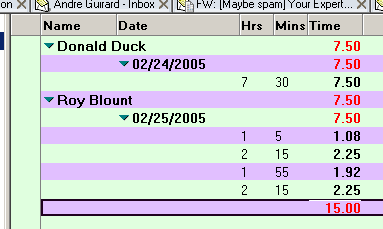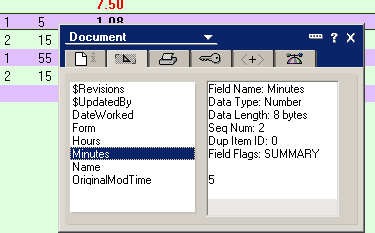Columns displaying wrong decimal from calculation

I have a view that displays imported employees attendance documents from a .txt file. This view has columns, a couple of which are hours and minutes. When I try calculating hours + minutes for each individual employee, I get the correct total figure in decimal format, just a little off. For example, if an employee worked 7.5 hours, the column will display 7.67.

How can I get the column to display 7.5 hours instead? The formula that I am using in the column is:

@Sum(minutes)/60 + @Sum(Hours)
Your help with this is greatly appreciated.
Assuming the Hours field contains 7 and the Minutes field contains 30, I see no reason the result should be 7.67. Are you sure the Minutes field doesn't contain 40?

I don't understand why you use @Sum. Are these multivalued fields? If not, @Sum doesn't do anything useful. If so, then what values are you using to represent 7.5 hours?

If the fields are multivalued and Minutes contains, say, 30 and 10, then the formula is returning the correct result.

Use the document properties box from a view (highlight a document and press Alt+Enter) to see the actual values of these fields (on the second tab of the dialog). It might be that they don't have exactly the values that you think they do.

Member clarification:
Andre, first I would like to thank you for taking the time to read and answer my e-mail. Somehow though, there was some misunderstanding that may have been caused by my explanation.

I have a form "TATC" and on that form I have many fields, among those fields are two field "hours" and "minutes." The value of all the fields on the forms is imported from a .txt file and saved into the document. As these documents are attendance documents, each employee will have at least four documents per day, in which case I need to total the amount of hours worked per that day. When I try to display the daily total @Sum(minutes)/60 + @Sum(Hours), which give me the minutes in decimal value as a portion of a hundred. I have no problem with the hours because as soon as the minutes portion hits 100% (equivalent to 60 minutes) then the hour is added to the hours.

My question was: Instead of the value of minutes display in decimal (portion of 100% of 60 minutes), I just want to display as is. For example, when an employee has worked 7 hours and 30 minutes, I would like to column to display 7.5 (.5 being half of the hour or 30 minutes from the 60 minutes of the hour).

I am so sorry for my lengthy explanation, and I hope that it help you to understand the problem better. I have tried many ways to go around the problem by using @int and integer functions and so on, but nothing worked.

Andre's response:
I still don't exactly understand what you're doing. I tried what I think you are describing, and I don't get 7.67 as a total. Perhaps I can explain to you what I did, and you can tell me how your situation is different.

I created a sample database that contains one form, Timecard, and one view. Each Timecard document contains one Hours number and one Minutes number.

The view contains a column with the formula @Sum(minutes)/60 + @Sum(Hours). The @Sum doesn't actually do anything useful, since there is only one number in each document to sum. So I could have used this formula instead, and it would do the same thing: Minutes/60 + Hours

Here's what the view looks like:Donald Duck worked 7 hours and 30 minutes, and the correct number 7.50 is displayed. This column also displays totals, so Roy Blount, who worked a total of 6 hours and 90 minutes stored in four separate documents, also has a correct total value 7.50 is displayed. I don't know where you're getting 7.67.

One thing you might want to do is examine the properties of the individual documents to make sure you know exactly what values they contain and what their datatypes are. Highlight a document in the view, and open the properties dialog by pressing Alt+Enter. Here's the tab that shows field information. Notice the Data Type of the field, which should be Number, and the value -- 5 in this case.Dig Deeper on Web Development for Lotus Notes Domino

• Favorite iSeries cheat sheets

Here you'll find a collection of valuable cheat sheets gathered from across the iSeries/Search400.com community. These cheat ...

Close